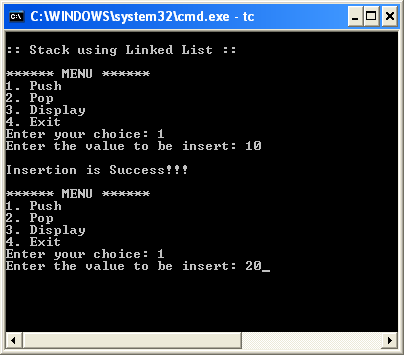The perfect place for easy learning...

# Data Structures

×

## Topics List

The major problem with the stack implemented using an array is, it works only for a fixed number of data values. That means the amount of data must be specified at the beginning of the implementation itself. Stack implemented using an array is not suitable, when we don't know the size of data which we are going to use. A stack data structure can be implemented by using a linked list data structure. The stack implemented using linked list can work for an unlimited number of values. That means, stack implemented using linked list works for the variable size of data. So, there is no need to fix the size at the beginning of the implementation. The Stack implemented using linked list can organize as many data values as we want.

In linked list implementation of a stack, every new element is inserted as 'top' element. That means every newly inserted element is pointed by 'top'. Whenever we want to remove an element from the stack, simply remove the node which is pointed by 'top' by moving 'top' to its previous node in the list. The next field of the first element must be always NULL.

# ExampleIn the above example, the last inserted node is 99 and the first inserted node is 25. The order of elements inserted is 25, 32,50 and 99.

# Stack Operations using Linked List

To implement a stack using a linked list, we need to set the following things before implementing actual operations.

• Step 1 - Include all the header files which are used in the program. And declare all the user defined functions.
• Step 2 - Define a 'Node' structure with two members data and next.
• Step 3 - Define a Node pointer 'top' and set it to NULL.
• Step 4 - Implement the main method by displaying Menu with list of operations and make suitable function calls in the main method.

# push(value) - Inserting an element into the Stack

We can use the following steps to insert a new node into the stack...

• Step 1 - Create a newNode with given value.
• Step 2 - Check whether stack is Empty (top == NULL)
• Step 3 - If it is Empty, then set newNode → next = NULL.
• Step 4 - If it is Not Empty, then set newNode → next = top.
• Step 5 - Finally, set top = newNode.

# pop() - Deleting an Element from a Stack

We can use the following steps to delete a node from the stack...

• Step 1 - Check whether stack is Empty (top == NULL).
• Step 2 - If it is Empty, then display "Stack is Empty!!! Deletion is not possible!!!" and terminate the function
• Step 3 - If it is Not Empty, then define a Node pointer 'temp' and set it to 'top'.
• Step 4 - Then set 'top = top → next'.
• Step 5 - Finally, delete 'temp'. (free(temp)).

# display() - Displaying stack of elements

We can use the following steps to display the elements (nodes) of a stack...

• Step 1 - Check whether stack is Empty (top == NULL).
• Step 2 - If it is Empty, then display 'Stack is Empty!!!' and terminate the function.
• Step 3 - If it is Not Empty, then define a Node pointer 'temp' and initialize with top.
• Step 4 - Display 'temp → data --->' and move it to the next node. Repeat the same until temp reaches to the first node in the stack. (temp → next != NULL).
• Step 5 - Finally! Display 'temp → data ---> NULL'.

# Implementation of Stack using Linked List | C Programming

``````#include<stdio.h>
#include<conio.h>

struct Node
{
int data;
struct Node *next;
}*top = NULL;

void push(int);
void pop();
void display();

void main()
{
int choice, value;
clrscr();
printf("\n:: Stack using Linked List ::\n");
while(1){
printf("1. Push\n2. Pop\n3. Display\n4. Exit\n");
scanf("%d",&choice);
switch(choice){
case 1: printf("Enter the value to be insert: ");
scanf("%d", &value);
push(value);
break;
case 2: pop(); break;
case 3: display(); break;
case 4: exit(0);
default: printf("\nWrong selection!!! Please try again!!!\n");
}
}
}
void push(int value)
{
struct Node *newNode;
newNode = (struct Node*)malloc(sizeof(struct Node));
newNode->data = value;
if(top == NULL)
newNode->next = NULL;
else
newNode->next = top;
top = newNode;
printf("\nInsertion is Success!!!\n");
}
void pop()
{
if(top == NULL)
printf("\nStack is Empty!!!\n");
else{
struct Node *temp = top;
printf("\nDeleted element: %d", temp->data);
top = temp->next;
free(temp);
}
}
void display()
{
if(top == NULL)
printf("\nStack is Empty!!!\n");
else{
struct Node *temp = top;
while(temp->next != NULL){
printf("%d--->",temp->data);
temp = temp -> next;
}
printf("%d--->NULL",temp->data);
}
}```
```

Output